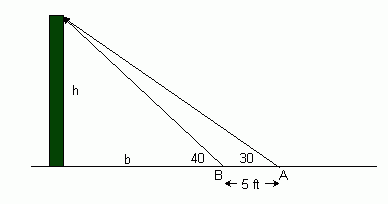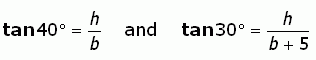SEARCH HOMEMath Central Quandaries & QueriesQuestion from joyce, a student: An observer wishes to determine the height of a tower. He takes sight @ the top of the tower from A & B w/c are 5oft. apart @ the elevation on a direct line w/ the tower. The vertical angle @ point A is 30 degrees & the point B is 40 degrees. What is the height of the tower? Find the value of x in angle tangent 40 degrees and 30 degrees? Show the solution of the value of x?Hi Joyce. I can get you started.

Here is a diagram of the question. You are asked to find the height I've marked as h in the diagram.Using the tangent function, we can see thatHere are two equations with two variables. Do you remember how to finish the algebra and solve for h?

Hope this helps,
Stephen La RocqueMath Central is supported by the University of Regina and The Pacific Institute for the Mathematical Sciences.